## Example Questions

### Example Question #22 : Trigonometry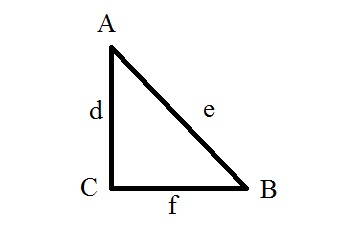For triangle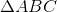, what is the cotangent of angle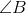?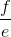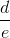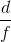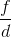Explanation:

The cotangent of the angle of a triangle is the adjacent side over the opposite side. The answer is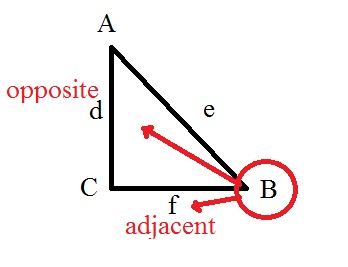### Example Question #23 : Trigonometry

What is the tangent of the angle formed between the origin and the point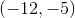if that angle is formed with one side of the angle beginning on the-axis and then rotating counter-clockwise to? Round to the nearest hundredth.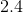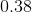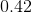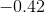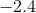Explanation:

Recall that when you calculate a trigonometric function for an obtuse angle like this, you always use the-axis as your reference point for your angle. (Hence, it is called the "reference angle.")

Now, it is easiest to think of this like you are drawing a little triangle in the third quadrant of the Cartesian plane. It would look like: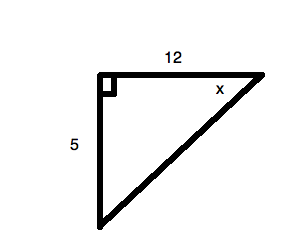So, the tangent of an angle is: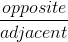or, for your data,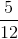This is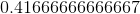. Rounding, this is.  Sinceis in the third quadrant, your value must be positive, as the tangent function is positive in that quadrant.

### Example Question #24 : Trigonometry

What is the tangent of the angle formed between the origin and the point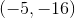if that angle is formed with one side of the angle beginning on the-axis and then rotating counter-clockwise to? Round to the nearest hundredth.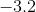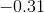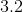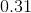Explanation:

Recall that when you calculate a trigonometric function for an obtuse angle like this, you always use the-axis as your reference point for your angle. (Hence, it is called the "reference angle.")

Now, it is easiest to think of this like you are drawing a little triangle in the third quadrant of the Cartesian plane. It would look like: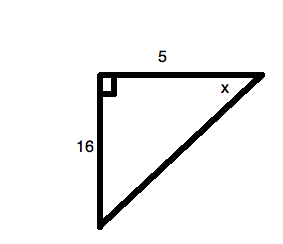So, the tangent of an angle is:or, for your data,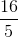, or. Sinceis in the third quadrant, your value must be positive, as the tangent function is positive in this quadrant.

### Example Question #25 : Trigonometry

A ramp is being built at an angle offrom the ground. It must coverhorizontal feet. What is the length of the ramp? Round to the nearest hundredth of a foot.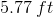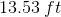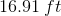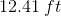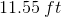Explanation:

Based on our information, we can draw this little triangle: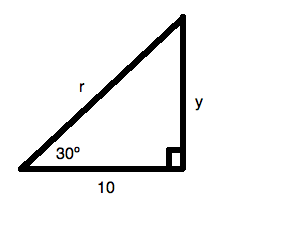Since we know that the tangent of an angle is, we can say: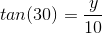This can be solved using your calculator: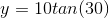or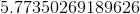Now, to solve for, use the Pythagorean Theorem,, whereandare the legs of a triangle andis the triangle's hypotenuse. Here,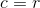, so we can substitute that in and rearrange the equation to solve for: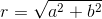Substituting in the known values: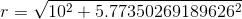, or approximately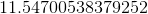. Rounding, this is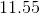.